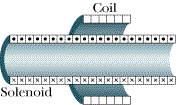# Inductance of Solenoid on Coaxial Cable

## Homework Statement

The figure below shows a short coil which is coaxial with a very long solenoid (you may approximate it as infinite.)

Coil: Has 120 turns of radius 1.8 cm and resistance 5.8 . It is not attached to anything.
Solenoid: Has 22700 turns/m and a radius of 1.6 cm. It receives an external current (source not shown).a.) The magnetic field across the coil's entire cross-sectional area (as caused by the solenoid) at any given time: ?

b.)
If the current supplied to the solenoid drops at a constant rate from 1.5 A to zero in in a time interval Δt = 25 ms, What are the magnitudes of the emf and current induced in the coil during this time interval?

c.) What is the mutual inductance of this solenoid/coil system?

## Homework Equations

Mutual inductance of coil 2 with respect to coil 1 = M12 = ( N2 * flux12 ) / I1
Mutual-induced emf by 1 on 2 = ε2 = - M * d/(dt) * I1
Bsolenoid = μoNI/l
flux = integral of B dot dA

## The Attempt at a Solution

For part (a):
Is a non-zero constant inside the solenoid and zero everywhere else?

For part (c):
I get the mutual inductance, M, is equal to ( μo * N1 * N2 * Area ) / length, however I'm not given a length.

## The Attempt at a Solution

Last edited:

rude man
Homework Helper
Gold Member

Let"1" refer to the solenoid and "2" refer to the coil.

You have B1 = μ0n1i1 (well-known solenoid formula, n1 = no. of turns per meter)

You also have Farady's law: emf2 = -N2*d(flux2)/dt
so what is flux2 in terms of B1?

Then substitute B1 in the eq. for emf2.

You further have, by definition,
emf2 = M di1/dt, M = mutual inductance.
So now solve for M.

## The Attempt at a Solution

For part (a):
Is a non-zero constant inside the solenoid and zero everywhere else?
Constant?

so what is flux2 in terms of B1?

flux2 = n2 * B1 * A1, where A1 is cross-sectional area of the solenoid.

Thanks! Got that part right, now working on the rest.

Last edited:
rude man
Homework Helper
Gold Member
flux2 = n2 * B1 * A1, where A1 is cross-sectional area of the solenoid.

Thanks! Got that part right, .

No, I don't think you did.
Isn't flux always some B times some A? What does N or n have to do with it?

No, I don't think you did.
Isn't flux always some B times some A? What does N or n have to do with it?

Oops, you're right. flux2 should just be B1 * A1. Though this is where I'm getting confused right now. On my equation sheet, provided by the instructor, our equation for magnetic flux looks like the following: flux = N * Integral ( B dot dA ) :/

In the end [of that part of the problem] I got emf2 = ( μo * N2 * N1 * Ii * A1 ) / Δt, where Ii is the initial current (1.5A), A1 is the cross-sectional area of the solenoid, and Δt is 0.025s. It came out to 165.18mV, which was marked correct.

Last edited:
Constant?
Recall from the problem, it states: "very long solenoid (you may approximate it as infinite.)"

So I have four choices for part a, which asks, The magnetic field across the coil's entire cross-sectional area (as caused by the solenoid) at any given time ...
1) is zero accross the entire area
2) is a constant non-zero value across the entire area
3) is a zero value in some locatoins an a constant non-zero value in others
4) varies continuously across the entire area.

From what I've read, I'm thinking the magnetic field outside the solenoid is zero, and that inside the solenoid it is pretty much constant.

rude man
Homework Helper
Gold Member
Oops, you're right. flux2 should just be B1 * A1. Though this is where I'm getting confused right now. On my equation sheet, provided by the instructor, our equation for magnetic flux looks like the following: flux = N * Integral ( B dot dA ) :/
You have to look at the context. Maybe he/she meant that the flux due to N windings in a solenoid is N times the flux due to 1 winding, etc. But flux = ∫B*dA always.
In the end [of that part of the problem] I got emf2 = ( μo * N2 * N1 * Ii * A1 ) / Δt, where Ii is the initial current (1.5A), A1 is the cross-sectional area of the solenoid, and Δt is 0.025s. It came out to 165.18mV, which was marked correct.

Your N1 should be n1, no. of turns per meter. But that's what you obviously used to get the right answer. Just remember the distinction ...rude man
Homework Helper
Gold Member
Recall from the problem, it states: "very long solenoid (you may approximate it as infinite.)"

So I have four choices for part a, which asks, The magnetic field across the coil's entire cross-sectional area (as caused by the solenoid) at any given time ...
1) is zero accross the entire area
2) is a constant non-zero value across the entire area
3) is a zero value in some locatoins an a constant non-zero value in others
4) varies continuously across the entire area.

From what I've read, I'm thinking the magnetic field outside the solenoid is zero, and that inside the solenoid it is pretty much constant.

That's what I'm thinking!